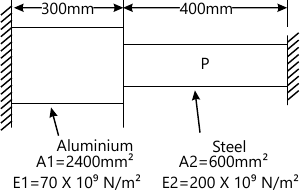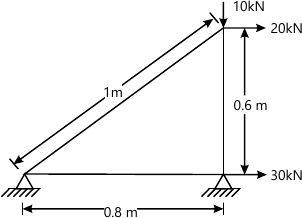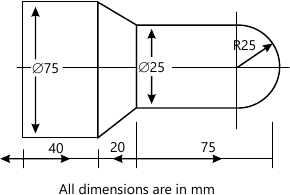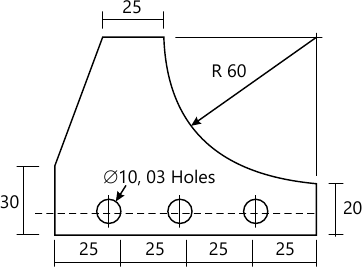SPPU Mechanical Engineering (Semester 7)
May 2014
Total marks: --
Total time: --
INSTRUCTIONS
(1) Assume appropriate data and state your reasons
(2) Marks are given to the right of every question
(3) Draw neat diagrams wherever necessary

Answer any one question from Q1 and Q2
1 (a) Define and compare Geometric Mappings and Geometric Transformations.
4 M
1 (b) Calculate the concatenated transformation matrix, if the transformations are performed in the following sequence.
i) Translation through 4 and 5 units along X and Y directions respectively.
ii) Scale by a factor of 2 and 4 units in X and Y directions respectively.
iii) Rotation by 60° in CCW direction about Z-axis passing through point (4, 4). What will be effect of above transformations on a triangle ABC with A (4, 4), B(8,4), and C (6, 8). Sketch the original triangle and triangle after transformation.
12 M

2 (a) Explain how orthographic Top view and Front view are obtained in CAD software by proper transformation mapping.
6 M
2 (b) A triangle with vertices P(2, 4), Q(4, 6) and R(2, 6) is to be reflected about the line y = 0.5x+2. Determine,
i) Composite Transformation Matrix.
ii) New vertices of triangle PQR.
10 M

Answer any one question from Q3 and Q4
3 (a) Explain the concept of hybrid modelling.
6 M
3 (b) Line L1 has end points (l,2,7) and (5,6,1),while line L2 has end points (7,3,4) and (3,9,10).
i) Find the parametric equation of the lines.
ii) Find the tangent vectors of the lines.
iii) Are the two lines parallel or perpendicular?
iv) Are the two lines intersecting? If yes, find the point of intersection.
10 M

4 (a) Distinguish between analytical and synthetic curves.
5 M
4 (b) Explain the feature based modelling technique.
5 M
4 (c) Find the degree of the Bezier curve controlled by three points (4, 2), (0, 0) and (2, 8). Also find the equation of Bezier curve in parametric format with parameters 'u'.
6 M

Answer any one question from Q5 and Q6
5 (a) Explain the significance of the shape functions for 1D two node element.
6 M
5 (b) Consider the bar shown in Fig 1 is subjected to axial load P =200 X 103N. Using the penalty approach for handling boundary conditions,do the following:
i) Determine the nodal displacements. ii) Determine the stress in each material.
iii) Determine the reaction forces.12 M

6 (a) Derive the relation between natural and local coordinate system for two nodded 1D bar element.
6 M
6 (b) The three-bar truss made of steel (E=200 X 103 N/mm2 ) is subjected to the horizontal forces of 30kN and 20kN and the vertical forces of l0kN,as shown in the Fig. 2.The cross-sectional area is 300 mm2 for each element. Using the finite element method.
Determine:
i) The nodal displacement;
ii) The stresses in each element; and
iii) The reaction forces at the supports.12 M

Answer any one question from Q7 and Q8
7 (a) Write CNC part program to turn a mild steel bar of size and shape as shown in Fig. 3. Use canned cycles only for both rough turning and finish cut.Take feed rate of 0.5 mm/rev and spindle speed is equal to 200 RPM.12 M
7 (b) Classify CNC Machines based on:
1. Motion type
2. Control loops
3. Number of axes
3 M
7 (c) Explain with neat sketch 2, 2 1?2 and 3 axes of CNC machines.
3 M

8 (a) Write a CNC part program to manufacture the component as shown in Fig. 4, assume suitable data for feed and speed. Use peck drill canned cycle for drilling operations and use right cutter radius compensation. Take thickness of the plate is 20mm.12 M
8 (b) Explain Direct Numerical Control and enlist its advantages and limitations over CNC.
6 M

Answer any one question from Q9 and Q10
9 (a) What is FMS and describe briefly the various FMS layouts.
8 M
9 (b) What are various work transfer mechanisms? Explain GENEVA mechanism with neat sketch.
8 M

10 (a) Define automation? Compare fixed, programmable and flexible automation systems.
8 M
10 (b) What do you understand by group technology? Explain any one method in detail for grouping the parts into part families.
8 M

Answer any one question from Q11 and Q12
11 (a) Explain with neat sketch work envelope of cylindrical and SCARA Robot configuration.
8 M
11 (b) Explain with neat sketches the use of robot in welding and material handling.
8 M

12 (a) Define industrial robotics as per Robotic Industries Association and explain different degree of freedom available to in robot wrist.
8 M
12 (b) With a neat sketch, explain the use of magnetic grippers used in robot. Enlist their advantages and limitations.
8 M

More question papers from CAD/CAM Automation